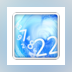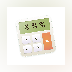### Percentages Calculator

Percentages Calculator is a perfect calculating tool for everybody, whether you are good at maths, or not.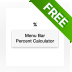Menu Bar Percent Calculator is a tiny menu bar application that helps you calculate percentages with ease.

... helps you calculate percentages with ease ... make percentages-related calculations instantly ...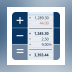### CalcTape Paper Tape Calculator

1 on 1 vote

Calculate. Comment. Correct. CalcTape is a revolutionary new kind of pocket calculator.

... any other percentage calculation easily. - ... , exponentials, percentage calculations and much ...### Scaletron!

Scaletron! is a scaling calculator — an electronic replacement for the old graphic arts proportion wheel ...

... and artwork, calculating page enlargements ... the scaling percentage. Enter an ...### Banking Finance Calculator

Banking Finance Calculator is an easy to use App that Contains 11 Financial Calculators

... Calculator includes the following Calculators: - Annual Percentage ...Imagine this scenario: You just scored individual questions on a stack of papers and now you need to calculate grades.

... it calculate the overall percentage, ... generated calculating the percentage corresponding ...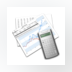### Stock Calculator

Stock Calculator is a profit/loss and share price calculator application.

... price calculator application. Stock Calculator is ... flat rate, percentage, per share ...### MacAppStuff Percent

Calculates the percentage of a figure, and shows the result along with the plus total (percentage added) and minus total (percentage deducted).

Calculates the percentage of a figure, ... minus total (percentage deducted). You ...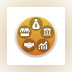### Finance Calculator

Finance Calculator is an easy to use App that Contains 97 Financial Calculators Split into 5 Categories:

... Includes 31 Calculators: - Annuity - ... Includes 11 Calculators: - Annual Percentage Yield ...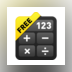### Calculator • Free

A simple calculator that will suit your everyday calculation needs. Calculate decimals, percentages & much more in this beautifully crafted top bar app.

... everyday calculation needs. Calculate decimals, percentages & much ...